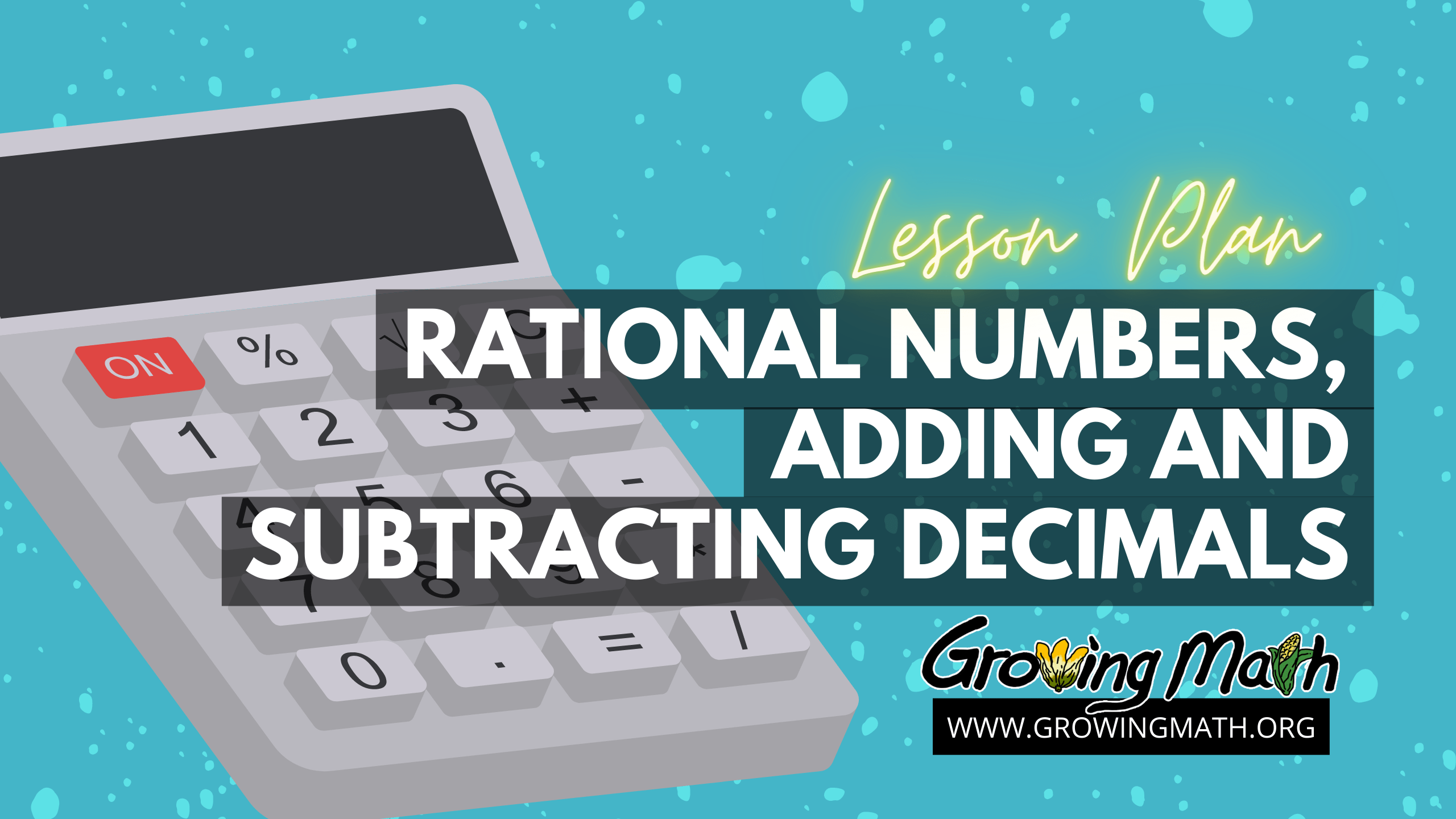# Tag Archives: decimals# Rational numbers, adding and subtracting decimals

## 📖STANDARD

CCSS.MATH.CONTENT.7.NS.A.1 Apply and extend previous understandings of addition and subtraction to add and subtract rational numbers

CCSS.MATH.CONTENT.7.EE.B.3 Solve multi-step real-life and mathematical problems posed with positive and negative rational numbers

30-45 minutes

## 📲TECHNOLOGY REQUIRED

A projector and computer in class or a computer, phone or tablet at home is required to watch the videos and see the Google slides. Slides can also be printed and sent home with students.

## 📃LESSON SUMMARY

Students watch two videos explaining decimal and fraction equivalence. They are then presented with a brief reminder of natural, integer and rational numbers. A slide presentation discusses adding and subtracting decimals. The lesson ends with teacher and student-generated practice problems.

## 📚LESSON

Since the previous lesson discussed rational numbers and fractions, we introduce this lesson with two videos explaining the equivalence of fractions and decimals.

### Video Introducing Decimals

This three minute video discusses fractions, decimals and percents as three ways of expressing the same number.

This second video shows examples of fractions and decimals as equivalent.

### Presentation and Practice Problems

Use this Google slides presentation to reinforce the understanding of rational numbers introduced in the previous lesson. Students can answer two formative assessment questions during a presentation or these can be done on their own with students learning at home. The lesson ends with students writing and sharing their own decimal addition and subtraction problems with classmates.

#### Enrichment Option

To provide motivated or advanced students more information on types of numbers, assign this optional two-page reading on types of numbers.

## ASSESSMENT

Formative assessment problems are given in the presentation. Students also write their own problems and answers. AzTech: Empiric Empire teacher reports are available for assessing student answers in Data and Reports.

### STATE STANDARD

Missouri Learning Standards (MLS) 7.NS.A.1.a